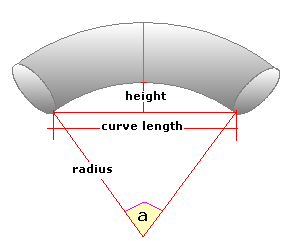Spike's Calculators

# Curved Pipe VolumeCalculate the volume of a curved section of pipe using length and the curve's height. If you don't know the angle of the curve or the bend radius, you can use this calculator to calculate the volume of a curved section of pipe.
This calculator uses inches for measurements.

### Volume from Curve Length and Height

Curve Length in
Curve Height in
Pipe Diameter in
Wall Thickness in
Decimal Precision #

#### Results:

Internal Angle of Curve °
###### Volume:
In Cubic Inches in³
In Cubic Feet ft³
In Cubic Metre
###### Pipe Capacity:
In US Gallons gal US
In Imperial Gallons gal Imperial
In Litres L

#### Calculator

1. enter the length of the curve in inches
2. the height of the curve in inches
3. the outside diameter of the pipe in inches
4. the thickness of the pipe wall in inches
5. decimal precision for the first two results

#### Results

1. the bend radius of the curve in inches
2. the internal angle in degrees
3. the volume of the curved section in cubic inches
4. the volume in cubic feet
5. the volume in cubic metres
6. the capacity of the curved section in US gallons
7. the capacity in Imperial gallons
8. the capacity in litres

### Formula

```V = 2.4674((d-t+r)²-(r+d)²)(d-2t)(a/360)
where V is the volume of the curve section
2.4674 a constant
d the pipe diameter
t the thickness of the pipe wall
r the radius of the curve
the interior angle of the curve
```

### Conversions

```one cubic inch (in³) = 0.000578703703703704 cubic feet (ft³)
one cubic inch (in³) = 0.000016387064 cubic metres (m³)
one cubic inch (in³) = 0.00432900432900432 gallons (gal) US
one cubic inch (in³) = 0.00360465014990905 gallons (gal) Imperial
one cubic inch (in³) = 0.016387064 litres (L)
```